Payal Tandon
Co-founder, e-GMAT
Welcome to e-GMAT Support!
I am Payal, Co-Founder of e-GMAT.
Feel free to ask any Query.We will be contacting you soon on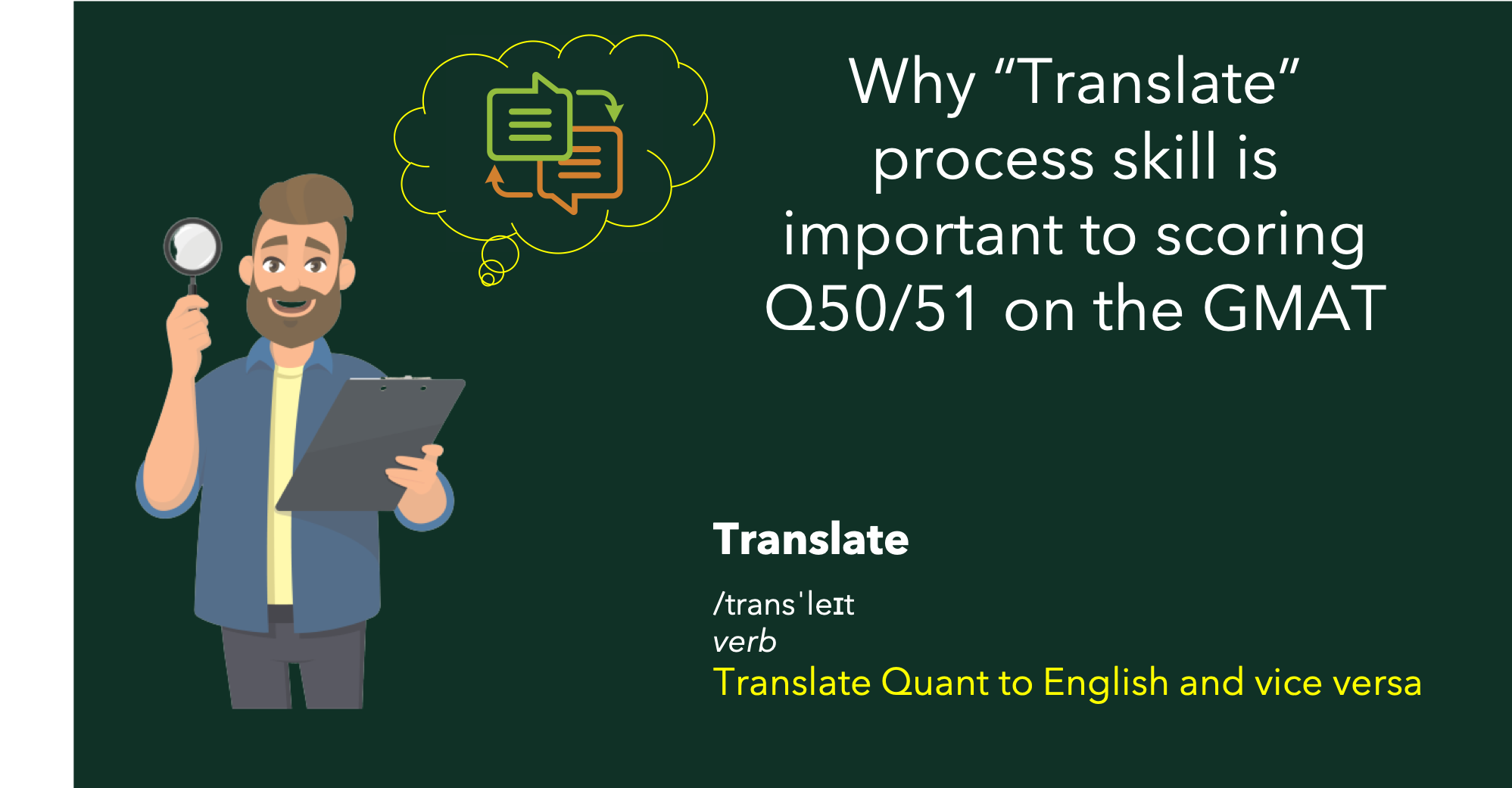As the name suggests, this process skill entails conversion of one language into another.  In the context of GMAT Quant questions, the two languages are English and Math.  Now to do the conversion, one definitely needs to know the acceptable constructs in the two languages.  So, you need to know the basics of the English language and you need to have the appropriate conceptual knowledge of the Math language.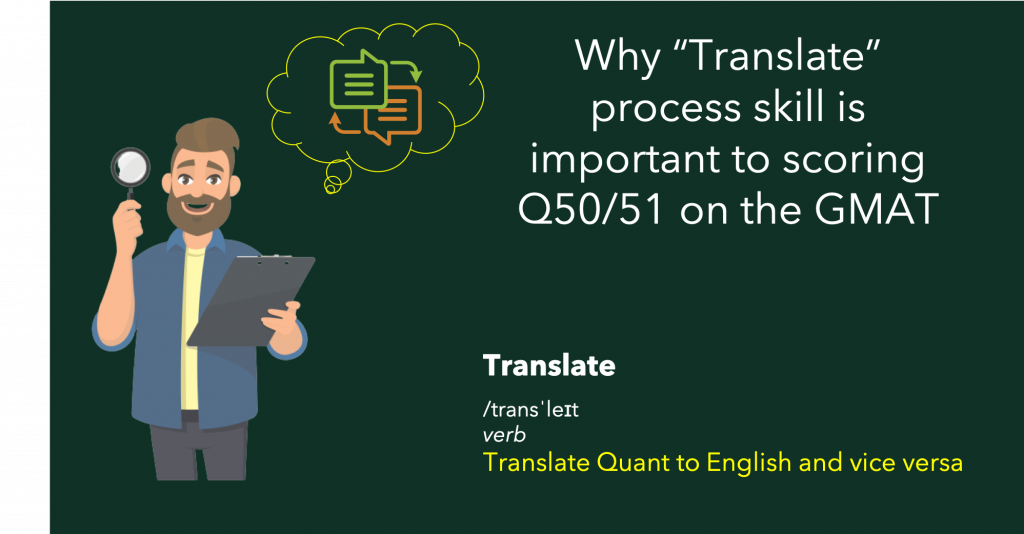Thus, as we discussed in the article introducing process skills, the definition of this process skill is as follows:

Converting English into Mathematical form and vice versa, utilizing your conceptual knowledge.

In case you haven’t gone through the first process skill, read it here.

Translate process skill is typically applied at the beginning of the solution to convert the given information from English to Math.  It may also be applied at the end of the solution depending on the format of the answer choices.  In fact, in certain cases, it may be applied in the middle of the solution as well.  So, when you begin reading the question statement, remind yourself to read it slowly so that you can translate the information appropriately.  As we will demonstrate through examples in this article, failure to properly apply translate process skill leads to a lack of clarity of how to approach the question, thereby not arriving at the correct answer.

In this article, we will show you how this process skill needs to be applied to quant questions.  For this demonstration, we have selected questions of varying difficulty levels from official sources from various sub-sections.  At the end of this article, we will give you a short quiz to test your skill set to translate information.

For the most effective experience, follow the sequence of steps below for each example:

1. Solve the question on your own.
2. Review the detailed solution.
3. Identify where you faltered if you faltered.
4. Read the analysis of the process skill.

Did you know a GMAT score of 730+ yields incremental \$500K in ROI? Start your GMAT Preparation by Signing up for our FREE Trial and get access to FREE online GMAT preparation resources. We are the most reviewed GMAT preparation company on GMATClub with more than 1870 reviews.

For the analysis of “translate” process skill, we will highlight the following aspects so that you not only observe how we applied this process skill on this question, but you also learn the framework of doing the same while solving questions on your own.

1. How did we break the sentence in order to “translate”?
2. What conceptual knowledge did we use to “translate”?
3. What was the translated statement?
4. How did the translated statement take us closer to the solution?

So, let’s get started with our first example.  This GMAT question is from the Number Properties topic.

Here’s a video explaining the 6 process skills

# Question 1 – Exemplifying the importance of “Translate”

This question is from Official Guide.  It is a relatively easier GMAT question, 600-level at best.  Solve this question on your own.

If [x] is the greatest integer less than or equal to x, what is the value of [-1.6] + [3.4] + [2.7]?

1. 3
2. 4
3. 5
4. 6
5. 7

Now review the solution.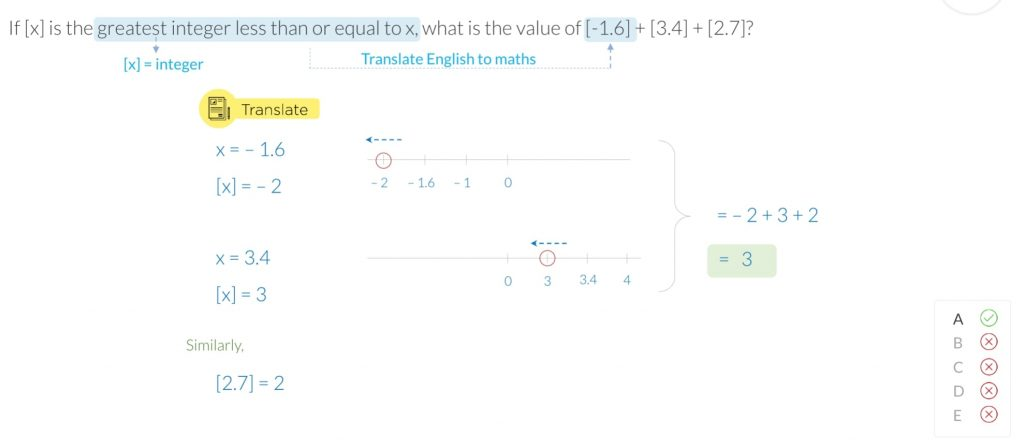We purposely selected this question so that you understand that you should apply this process skill on all questions and not just on difficult questions. In fact, students who deliberately apply these skills on easier questions, witness marked improvements while solving difficult questions as well.

Let’s look at the solution.  From the solution, it is very evident that the main feature of this question is the translation of information in the question statement.  So, let’s look at how we did the translation.

We read the question statement “If [x] is the greatest integer less than or equal to x, what is the value of [-1.6] + [3.4] + [2.7]?” slowly as follows. Notice how as we read a small part, we translated that part and then we put that together as we continued.

• If [x] is the greatest integer
• x is some number.
• [x] is an integer, i.e. it can be -ve or 0 or +ve.
• less than or equal to x,
• [x] is the greatest integer that is less than or equal to x.
• → it cannot be greater than x.
• That is “[x] is the greatest integer among the list of all integers that are less than or equal to the value of x”
• what is the value of [-1.6] + [3.4] + [2.7]?
• We need to find the sum of these terms.

Next, we took this definition of [x] and applied it on each term one by one.

• [-1.6]
• x = -1.6
• closest integer value that is less than -1.6 is -2.
• And -2 is the greatest integer that is less than -1.6.
• Thus, [-1.6] = -2

Now let’s translate [3.4] per the definition in the question statement.

• [3.4]
• x = 3.4
• closest integer value that is less than 3.4 is 3.
• And 3 is the greatest integer that is less than 3.4
• Thus, [3.4] = 3

And lastly, we will apply the definition on [2.7]

• [2.7]
• x = 2.7
• closest integer value that is less than 2.7 is 2.
• And 2 is the greatest integer that is less than 2.7
• Thus, [2.7] = 2

Notice carefully that our job of translation did not simply end after reading the question statement.  It also included application of that definition on the relevant terms in the question.

And now to finish off the solution, we simply do the calculation.

Before we move on to the next example, I want you to notice how we did the translation.  We read the question statement in small chunks and we translated as we read each small part.  And then we put it all together.  Essentially, we applied the process of strategic pausing that we use in verbal section of the GMAT.  😊

Also, in terms of our conceptual knowledge, we applied our understanding of order of integers – a very basic concept.

# Question 2 exemplifying the importance of “Translate”

Now solve this difficult 700-level question from the Official Guide.

Club X has more than 10 but fewer than 40 members. Sometimes the members sit at tables with 3 members at one table and 4 members at each of the other tables, and sometimes they sit at tables with 3 members at one table and 5 members at each of the other tables. If they sit at tables with 6 members at each table except one and fewer than 6 members at that one table, how many members will be at the table that has fewer than 6 members?

1. 1
2. 2
3. 3
4. 4
5. 5

And review its solution in detail.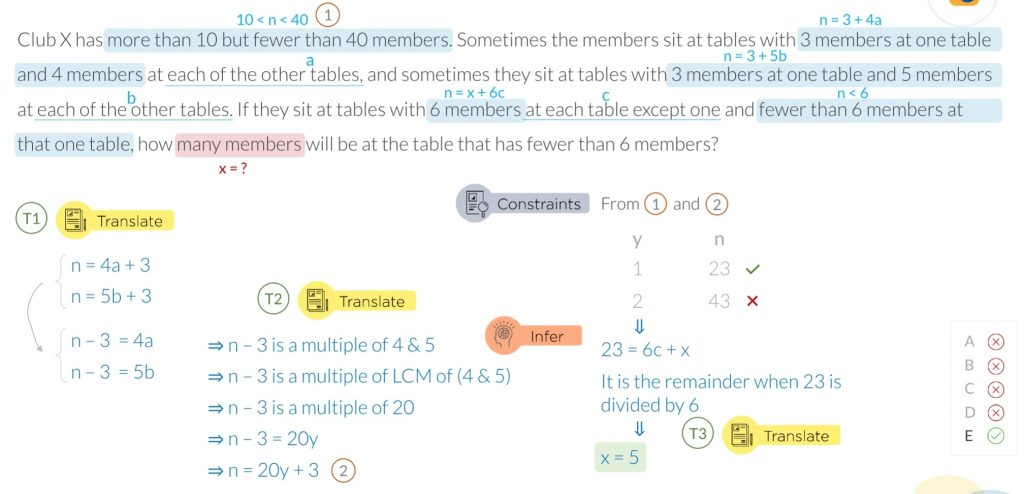Now let’s look at how we translated the information:

• Club X has more than 10 but fewer than 40 members.
• Let there be n members at Club X.
• 10 < n < 40
• Sometimes the members sit at tables with 3 members at one table and 4 members at each of the other tables,
• n = 4a + 3
• where a is the number of tables on which 4 people sit.
• and sometimes they sit at tables with 3 members at one table and 5 members at each of the other tables.
• n = 5b + 3
• where b is the number of tables on which 5 people sit.
• If they sit at tables with 6 members at each table except one and fewer than 6 members at that one table,
• n = 6c + x
• where
• c is the number of tables on which 6 people sit.
• x is the number of people on 1 table and x < 6
• how many members will be at the table that has fewer than 6 members?
• x = ?

This is the output of first instance of translation performed in this question.  We will write it down all together once again.

Output of 1st application of ‘translation’

1. 10 < n < 40
2. n = 4a + 3
3. n = 5b + 3
4. n = 6c + x
5. x = ?

Next, as we analyze the equations b and c, we observe and do second instance of translation as follows:

• n – 3 = 4a
• n – 3 is a multiple of 4
• n – 3 = 5b
• n – 3 is a multiple of 5

From this output, we infer as follows:

• n – 3 is a multiple of LCM of 4 and 5
• n – 3 is a multiple of 20
• n – 3 = 20y
• n= 20y + 3

And now in the last part of the solution, as we identify the possible values of n using the last equation and using the constraints in the question, we arrive at the conclusion that the only possible value of n is 23.

And this leads us to the last instance of translation in this question.

• 23 = 6c + x

We translate this equation as follows:

• x is the remainder when 23 is divided by 6.

And we arrive at the value of x as 5.

Now that we have seen the application of “translate” process skill, let’s look at the conceptual knowledge required to solve this question.

• Understanding of LCM
• Understanding of remainder equation

Before we move to our last example, let’s summarize the application of “translate” process skill in this question.

We did quite a bit of “translate” back and forth from English to Math and then from Math to English. And as we translated information, we understood the concepts that need to be applied.

• For example, after second application, we realized that we can apply the LCM concept.
• Likewise, after third application, we realized that we could use remainder concept to solve the question.

Essentially, by consciously applying process skills, you are encouraged to pause to assimilate information and that helps you to decide the path of the solution.  Or in other words, these process skills act as guiding light pointing to the solution of the question.

# Question 3 exemplifying the importance of “Translate”

Solve this GMAT Geometry ‘difficult’ 700-level question.  This question is from OG Advanced book.

A circular rim 28 inches in diameter rotates the same number of inches per second as a circular rim 35 inches in diameter. If the smaller rim makes x revolutions per second, how many revolutions per minute does the larger rim makes in terms of x?

1. 48π/x
2. 75x
3. 48x
4. 24x
5. x/75

And now review the solution.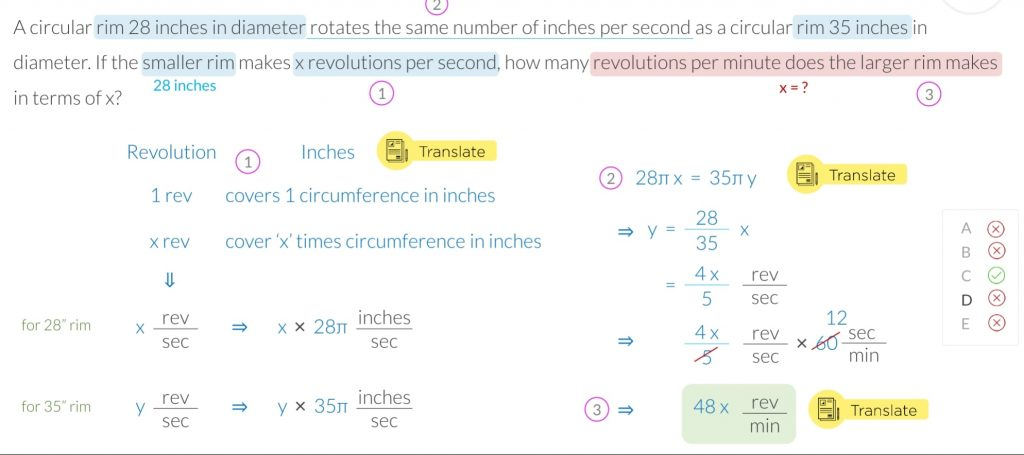Let’s read the question statement slowly by strategically pausing and translate the information as we read it.

• A circular rim 28 inches in diameter
• rim 1 = 28” dia
• Rotates the same number of inches per second
• same speed.
• As a circular rim 35 inches in diameter.
• rim 2 = 35” dia
• If the smaller rim makes x revolutions per second,
• rim 1 goes at x revolutions per second
• How many revolutions per minute does the larger rim make in terms of x?
• Need to find revolutions per second of rim 2.
• Note the units – revolutions per minute.

Now this question statement is very dense, and you may notice that we haven’t really translated a whole lot – i.e. our translated information so far does not look very different from the given question statement.  And that is by design.  Since the question statement is very dense, we read through it once assimilating some information and now we will browse through it again with a fine-toothed comb and analyze each part one by one.  This means that translation does not necessarily happen in one go.  At times, it’s an iterative process.

Let’s take another stab at it.  But this time, we will pick up from the above analysis.

Notice that for rim 1, we are given information about its speed in terms of revolutions per second.

So, let’s translate that further, while applying our understanding of circumference as follows:

• 1 revolution = 1 circumference = πd inches
• x revolution = xπd inches
• Thus, rim 1 covers 28πx inches in x revolutions

Now we can write this relationship for rim 2 as well.  But since we do not know the revolutions per second for rim 2 (that is what we need to find), let’s assume that to be y revolutions per second.

• Thus, rim 2 covers 35πy inches in y revolutions.

So, this was the complete translation of this part of the question statement:

A circular rim 28 inches in diameter rotates the same number of inches per second as a circular rim 35 inches in diameter. If the smaller rim makes x revolutions per second, how many revolutions per minute does the larger rim makes in terms of x?

Now next, we will translate the yellow highlighted part of the question statement by using the translation done so far.  And we arrive at the following equation:

• 28πx = 35πy

And now we do further processing of this equation as shown in the solution and we arrive at the following relationship.

• y = 4x/5 revolutions per second

And now we arrive at the last application of translation.  In the initial read of the question statement, we read that the required units are revolutions per minute.

And hence we need to convert the units and arrive at the following answer – 48x revolutions per minute.

Review the iterative process of “translate” once again and notice how we arrived at the answer by slowly processing the information.  And for the sake of completeness, let’s write down the conceptual knowledge required for this question:

1. Circumference of a circle
2. Unit conversion

# Concluding Remarks

Through these three examples, we have seen the various aspects of the application of “translate” process skill.  At a very high level, we know that:

1. It’s applicable to every type of question – easy or difficult.
2. It’s applicable on questions from any topic.
3. We need to apply our conceptual knowledge to translate information.

Through example 2 specifically, we learned that we may translate back and forth between English to Math throughout the solution and doing so guides us through the solution by giving us ideas of what concepts we need to apply.

And lastly, through example 3, we learned that the use of translate process skill as an iterative process.  The question may not be framed in such a way that we linearly process the information.  And that is why in those cases, we should think of this skill to be an iterative process.   Doing so helps us effectively chart out the solution to the question.

In summary, the operating principle behind the process skill of translate is simple – read the information slowly so that you give your brain sufficient time to assimilate the information properly so that you can chart your path to the solution of the question.#### Payal Tandon

###### Browse Related Topics# Achieve 740+on the GMAT in 30 days!Sign up for our free trial and get400+ Practice questions with detailed solutions10+ hours of AI-driven video lessonsAdaptive mock test with ESR+ analysis